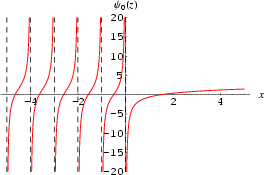•http://facebook.com/
•https://www.google.com/accounts/o8/id
•https://me.yahoo.comCOST (GBP)2.500.500

# Psi

viewed 2285 times and licensed 77 times
Psi (digamma) function
Controller: CodeCogsContents## DependentsC++
Excel

## Psi

 doublepsi( double x )
The Psi (also called the Digamma) function is a special function which is given by the logarithmic derivative of the gamma function. There is some ambiguity over its definition, as it is sometimes defined as the logarithmic derivative of the factorial. Due to this, two differet notiations are sometimes used, with the logarithmic derivative of the gamma function defined as:

And the logarithmic derivative of the factorial function defines as:

The two are connected by the relationshipPsi (Digamma) function Psi (digamma) function computes the logarithmic derivative of the gamma function:

For integer x, where EUL=0.57721566490153286061

This formula is used for 0 < x <= 10. If x is negative, it is transformed to a positive argument by the reflection formula

For general positive x, the argument is made greater than 10 using the recurrence .Then the following asymptotic expansion is applied:

where the are the Bernoulli numbers.

## Accuracy:

Relative error (except absolute when |psi| < 1): <pre> domain # trials peak rms -30,0 40000 1.5e-15 2.2e-16 </pre>

## Error Message:

psi singularity x integer <=0 MAXNUM

## References:

• Cephes Math Library Release 2.8: June, 2000
• http://mathworld.wolfram.com/DigammaFunction.html

### Example 1

#include <stdio.h>
#include <codecogs/maths/special/gamma/psi.h>

int main()
{
for(double x=-0.9; x<4; x+=0.2)
{
double y=Maths::Special::Gamma::psi(x);
printf("\n psi(%.1lf)=%lf", x,y);
}
return 0;
}
Output:
psi(-0.9)=-9.312644
psi(-0.7)=-2.073953
psi(-0.5)=0.036490
psi(-0.3)=2.113310
psi(-0.1)=9.245073
psi(0.1)=-10.423755
psi(0.3)=-3.502524
psi(0.5)=-1.963510
psi(0.7)=-1.220024
psi(0.9)=-0.754927
psi(1.1)=-0.423755
psi(1.3)=-0.169191
psi(1.5)=0.036490
psi(1.7)=0.208548
psi(1.9)=0.356184
psi(2.1)=0.485336
psi(2.3)=0.600040
psi(2.5)=0.703157
psi(2.7)=0.796783
psi(2.9)=0.882500
psi(3.1)=0.961526
psi(3.3)=1.034822
psi(3.5)=1.103157
psi(3.7)=1.167154
psi(3.9)=1.227328

### Parameters

 x input

### Authors

Stephen L. Moshier. Copyright 1984, 1987, 2000
Documented and Corrected by Will Bateman (August 2005)
##### Source Code

Source code is available when you agree to a GP Licence or buy a Commercial Licence.

Not a member, then Register with CodeCogs. Already a Member, then Login.